# php怎么从数组尾部增加元素php从数组尾部增加元素的方法

1、使用array_push()函数从数组尾部增加元素

array_push()函数可以在数组尾部插入一个或多个元素（键值），语法如下：

`array_push(\$array,\$value1,\$value2...)`

```<?php
\$arr=array(10,12,20);
array_push(\$arr,8,"9",3.14);
var_dump(\$arr);
?>```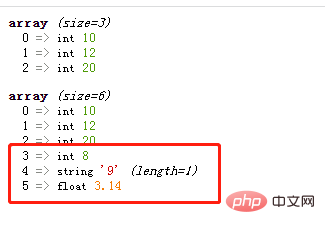array_push()函数插入的元素可以是数组类型

```<?php
\$arr=array(10,12,20);
var_dump(\$arr);
array_push(\$arr,array(1,2,3));
var_dump(\$arr);
?>```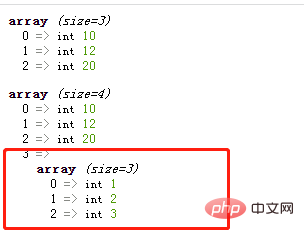2、使用array_splice()函数从数组尾部增加元素

`array_splice(\$array,\$start,\$length,\$value)`函数是一个强大的函数，可以用来删除数组元素、替换数组元素，也能用来插入数组元素（只需要将参数`\$length`的设置为0即可）。

`\$length=0`，那么参数`\$start`就可指定开始插入的位置（下标），参数`\$value`就可指定插入值（如果是多个值就需要设置为数组）。

```<?php
\$arr1=array(10,12,20);
array_splice(\$arr1,count(\$arr1),0,"1");
var_dump(\$arr1);

\$arr2=array(10,12,20);
array_splice(\$arr2,count(\$arr2),0,array("1",25,"3"));
var_dump(\$arr2);
?>```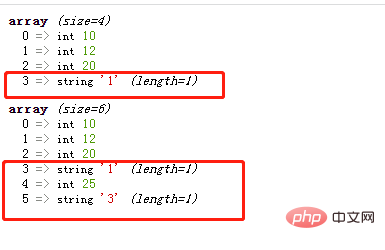`array_pad(\$array,\$size,\$value)`函数可以将某个键值`\$value`插入到数组`\$array`中，从而将数组填补到指定的长度`\$size`。（`\$size`参数可以理解为数组中元素的最终数目，即插入操作后的数组长度）。

• 当\$size参数为正数，则在数组尾部插入元素。

```<?php
\$arr=array(10,12,20);
var_dump(\$result);
?>```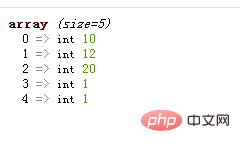```<?php
\$arr=array(10,12,20);
var_dump(\$result);
?>```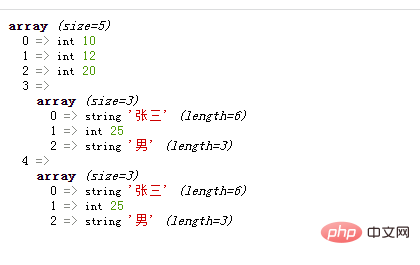4、使用array_merge()函数从数组尾部增加元素

`array_merge(array1,array2,array3...)` 函数用于把一个或多个数组合并为一个数组，将`array2,array3...`的值存入`array1`中。

```<?php
\$arr=array(10,12,20);
\$result =array_merge(\$arr,array(2,3,4)) ;
var_dump(\$result);
?>```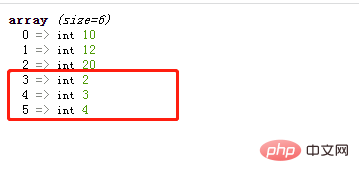1/1